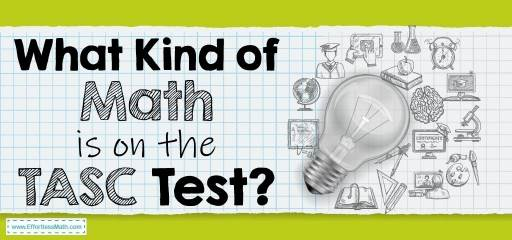# What Kind of Math Is on the TASC Test?The TASC (Test Assessing Secondary Completion™) is used as an updated alternative to other high school equivalence tests such as the GED. Passing the TASC standard test is equivalent to obtaining a high school diploma. Test takers can enter the university or find a better job by obtaining this diploma.

The TASC test consists of 5 subject area tests: Reading, Writing, Social Studies, Science, Mathematics.

The TASC Math test consists of two parts. The first section contains 40 multiple-choice questions that allow the use of a calculator. Test takers have 55 minutes to complete this section. The second part of the TASC test includes 12 Gridded-Response questions that the use of calculators is not allowed in this section. Participants in the test have 50 minutes to answer the questions of the second part.

The TASC Math test covers the following topics:

• Numbers and Quantities
• Algebra
• Functions
• Geometry
• Statistics and Probability

## Numbers and Quantities

The concepts you need in this section to know are the properties of rational and irrational numbers, differentiate between quantity changes, and solve math problems. In this section, you must have an understanding of how quantities change to one another and can use units correctly to solve problems.

## Algebra

In this section, you need to know how to use algebraic rules to solve a linear equation. At this point, you should be able to calculate algebraic expressions as well as addition, subtraction, multiplication, and division of polynomials.

## Functions

In this section, you will be asked to identify and analyze the system of equations. The ability to interpret expressions to solve the system of equations in this section is very important.

## Geometry

Geometry is the part of mathematics that measures your knowledge of using geometric formulas to calculate values. You need to have appropriate geometric reasoning skills to be able to perform well in this area. You should be able to use the graphs provided as relevant information to solve problems.

## Statistics and Probability

Your quantitative reasoning skills will be assessed in this section. The ability to calculate subsets is one of the things you need to know in this part of the TASC math test. Questions about the possibility of an event are also included in this section.

## FAQs:

### Is the TASC Math test hard?

Yes, the TASC test is a little more difficult than the GED test. TASC Math test can be challenging, but with enough practice, you can pass this test successfully.

### How do I study for the TASC Math?

How you prepare for the TASC Math test is very important. You can use good prep books to prepare well for the TASC Math test. You can also use online practice tests for further practice after studying the TASC exam courses.

## More from Effortless Math for TASC Test …

### You aren’t able to make time or achieve enough resources for being ready for the TASC math test?

You could get help from a variety of Top 10 Free Websites for TASC Math Preparation that we introduce to you.

Perhaps you might ask yourself Which Test Should You Take: GED, TASC, or HiSET? To answer your question, read the following article to find the difference between these tests, including their benefit and which test you should take between GED, TASC, AND HiSET.

You will have the chance to try out the most common TASC Math questions by using our “FREE TASC Math Practice Test” and “Full-Length TASC Math Practice Test“.

## Have any questions about the TASC Test?

### What people say about "What Kind of Math Is on the TASC Test? - Effortless Math: We Help Students Learn to LOVE Mathematics"?

No one replied yet.

X
49% OFF

Limited time only!

Save Over 49%

SAVE \$34

It was \$69.99 now it is \$35.99